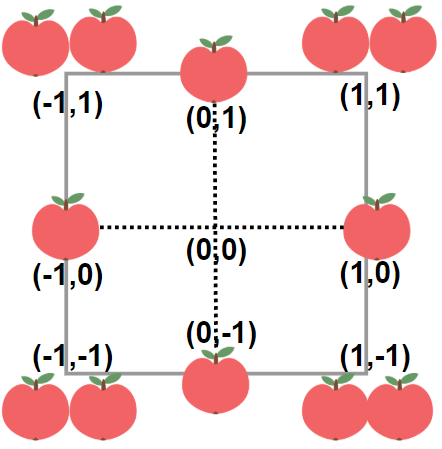# Minimum Garden Perimeter to Collect Enough Apples Solution Leetcode United states 2021

In a garden represented as an infinite 2D grid, there is an apple tree planted at every integer coordinate. The apple tree planted at an integer coordinate `(i, j)` has `|i| + |j|` apples growing on it.

You will buy an axis-aligned square plot of land that is centered at `(0, 0)`.

Given an integer `neededApples`, return the minimum perimeter of a plot such that at least `neededApples` apples are inside or on the perimeter of that plot.

The value of `|x|` is defined as:

• `x` if `x >= 0`
• `-x` if `x < 0`

Example 1:```Input: neededApples = 1
Output: 8
Explanation: A square plot of side length 1 does not contain any apples.
However, a square plot of side length 2 has 12 apples inside (as depicted in the image above).
The perimeter is 2 * 4 = 8.
```

Example 2:

```Input: neededApples = 13
Output: 16
```

Example 3:

```Input: neededApples = 1000000000
Output: 5040
```

Constraints:

• `1 <= neededApples <= 1015`